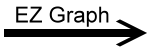﻿ EZ Graph - Tangent Function, Vertical Scaling and Translation Variables | Zona Land Education

# EZ Graph - Tangent Function, Vertical Scaling and Translation Variables

Click the 'Draw graph' button in the lower left corner of the EZ Graph app. Explanation below.

Here is the conventional notation for these tangent functions and the translation to the computer language type of notation used with EZ Graph:

 f1(x) = (a)tan(x - h) + kf1(x) = a * tan(x - h) + k f2(x) = tan(x)f2(x) = tan(x)

Notice that conventional notation does not require a multiplication operator (between (a) and tan(x - h)) while the EZ Graph notation does (the * between a and tan(x - h)).

Also note that no parentheses are required to force precedence. Multiplication proceeds before addition automatically, and that's just what we want.

Custom Search